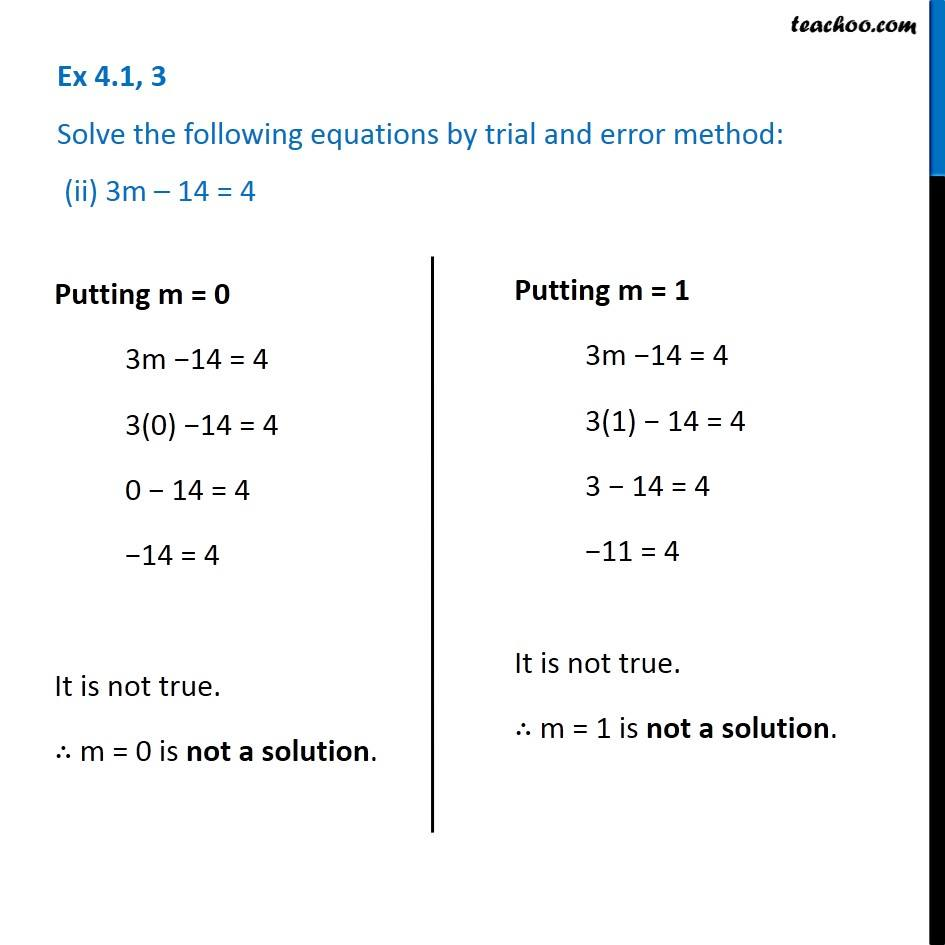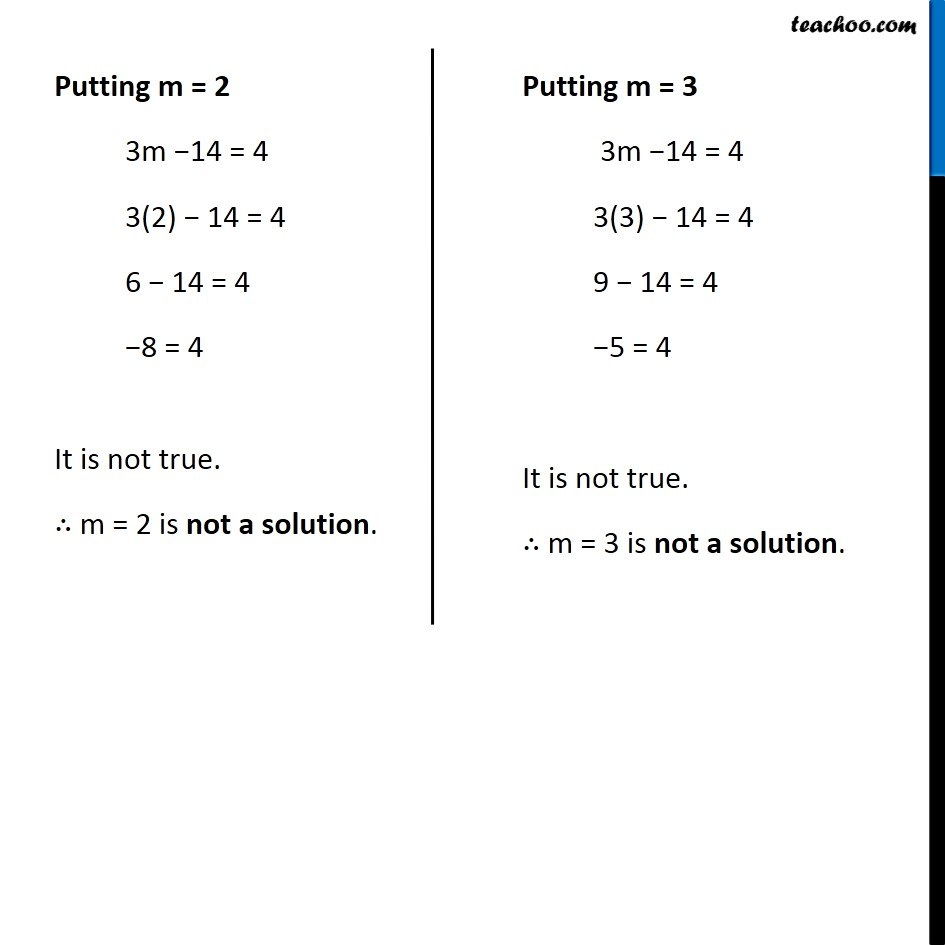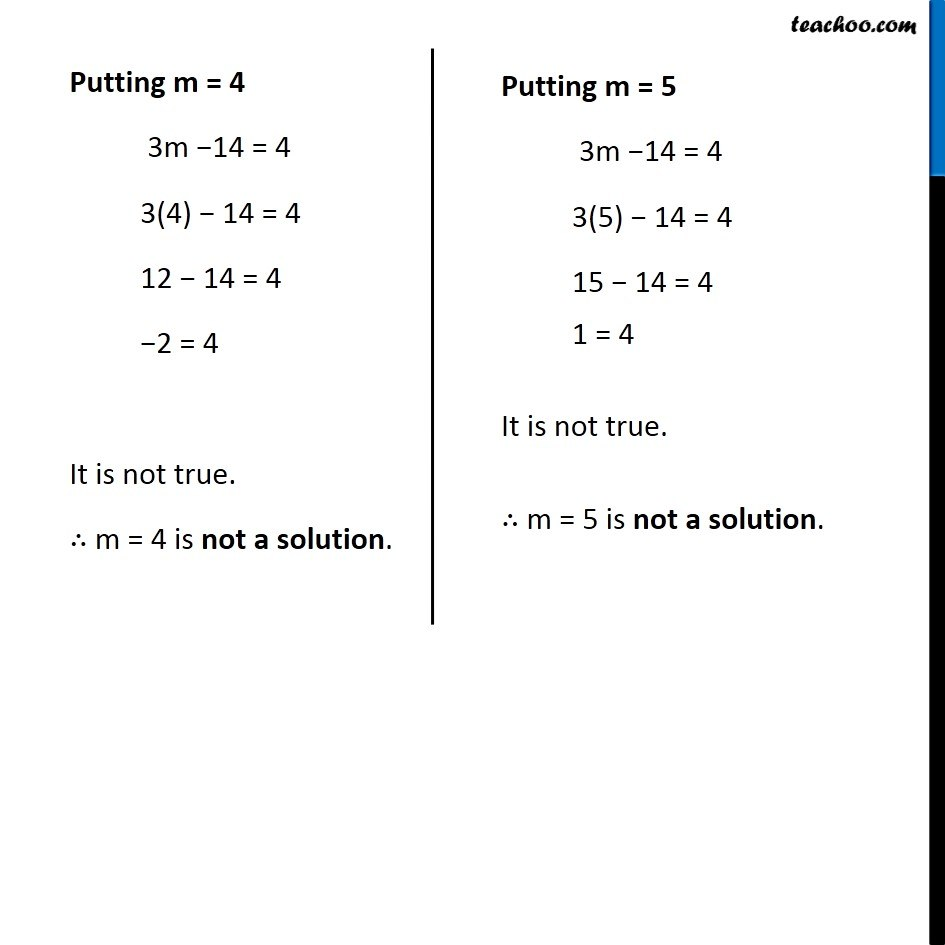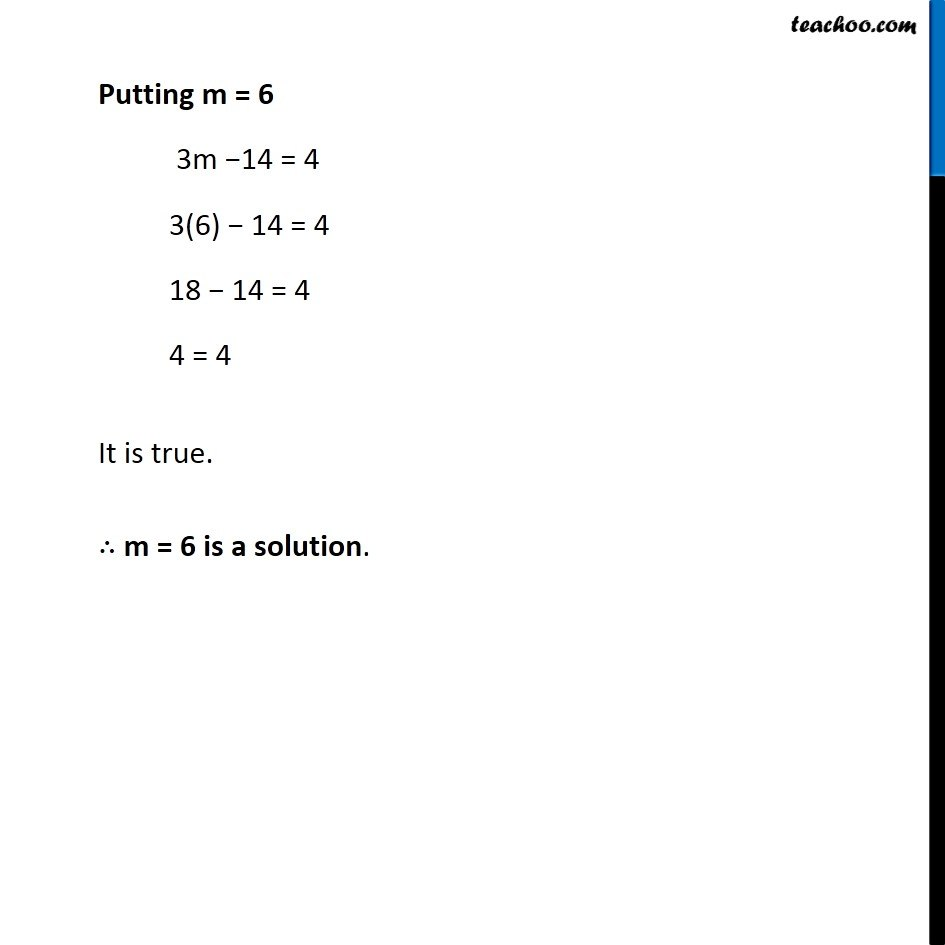Ex 4.1

Chapter 4 Class 7 Simple Equations
Serial order wiseLearn in your speed, with individual attention - Teachoo Maths 1-on-1 Class

### Transcript

Ex 4.1, 3 Solve the following equations by trial and error method: (ii) 3m – 14 = 4 Putting m = 0 3m −14 = 4 3(0) −14 = 4 0 − 14 = 4 −14 = 4 It is not true. ∴ m = 0 is not a solution. Putting m = 1 3m −14 = 4 3(1) − 14 = 4 3 − 14 = 4 −11 = 4 It is not true. ∴ m = 1 is not a solution. Putting m = 2 3m −14 = 4 3(2) − 14 = 4 6 − 14 = 4 −8 = 4 It is not true. ∴ m = 2 is not a solution. Putting m = 3 3m −14 = 4 3(3) − 14 = 4 9 − 14 = 4 −5 = 4 It is not true. ∴ m = 3 is not a solution. Putting m = 4 3m −14 = 4 3(4) − 14 = 4 12 − 14 = 4 −2 = 4 It is not true. ∴ m = 4 is not a solution. Putting m = 5 3m −14 = 4 3(5) − 14 = 4 15 − 14 = 4 1 = 4 It is not true. ∴ m = 5 is not a solution. Putting m = 6 3m −14 = 4 3(6) − 14 = 4 18 − 14 = 4 4 = 4 It is true. ∴ m = 6 is a solution.